# Chapter 10 Self Test

## Finding the rate law and mechanism for A+B<=>C+D

The following data were reported for the reaction A + B goes to C + D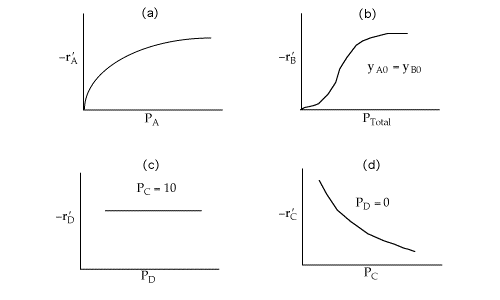Which of the following mechanisms is consistent with the above data?

## Solution

Recall

-r'A=-r'B=r'C=r'D

Figure (a) suggests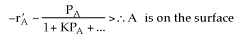Figure (b) suggests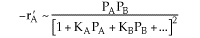Figure (c) suggests

-rA is not a function of PD, therefore the reaction is irreversible and D is not on the surface.Figure (d) suggests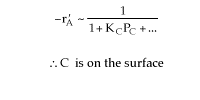Combining all the above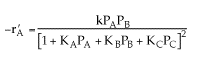Therefore, (d)is consistent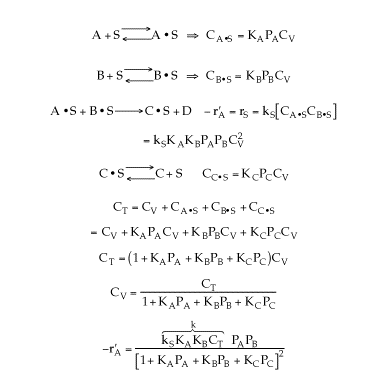Back to Chapter 10